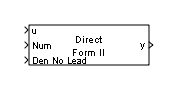Documentation

Transfer Fcn Direct Form II Time Varying

Implement time varying Direct Form II realization of transfer function

• Library:
• Simulink / Additional Math & Discrete / Additional DiscreteDescription

The Transfer Fcn Direct Form II Time Varying block implements a Direct Form II realization of the specified transfer function. The block supports only single input-single output (SISO) transfer functions.

The input signal labeled Den No Lead contains the denominator coefficients of the transfer function. The full denominator has a leading coefficient of one, but it is excluded from the input signal. For example, to use a denominator of [1 -1.7 0.72], specify a signal with the value [-1.7 0.72]. The input signal labeled Num contains the numerator coefficients. The data types of the numerator and denominator coefficients can be different, but the length of the numerator vector and the full denominator vector must be the same. Pad the numerator vector with zeros, if needed.

The block automatically selects the data types and scalings of the output, the coefficients, and any temporary variables.

Ports

Input

expand all

Input signal, specified as a scalar or vector.

Data Types: single | double | int8 | int16 | int32 | uint8 | uint16 | uint32 | Boolean | fixed point

Numerator coefficients of the transfer function, specified as a scalar or vector.

Dependencies

The data types of the numerator and denominator coefficients can be different, but the length of the numerator vector and the full denominator vector must be the same. Pad the numerator vector with zeros, if needed.

Data Types: single | double | int8 | int16 | int32 | uint8 | uint16 | uint32 | Boolean | fixed point

Denominator coefficients of the transfer function, specified as a scalar or vector, without the leading coefficient of one. The full denominator has a leading coefficient of one, but it is excluded from the input signal.

Example: For a denominator coefficient of [1 -1.7 0.72], specify a signal with value [-1.7 0.72].

Dependencies

The data types of the numerator and denominator coefficients can be different, but the length of the numerator vector and the full denominator vector must be the same.

Data Types: single | double | int8 | int16 | int32 | uint8 | uint16 | uint32 | Boolean | fixed point

Output

expand all

Output signal, specified as a scalar or vector.

The block automatically selects the data types and scalings of the output and any temporary variables.

Data Types: single | double | int8 | int16 | int32 | uint8 | uint16 | uint32 | Boolean | fixed point | enumerated | bus

Parameters

expand all

Specify the initial condition as a scalar.

Programmatic Use

 Block Parameter: vinit Type: character vector Values: scalar Default: '0.0'

Specify the rounding mode for fixed-point operations. For more information, see Rounding (Fixed-Point Designer).

Programmatic Use

 Block Parameter: RndMeth Type: character vector Values: 'Ceiling' | 'Convergent' | 'Floor' | 'Nearest' | 'Round' | 'Simplest' | 'Zero' Default: 'Floor'

When you select this check box, overflows saturate to the maximum or minimum value that the data type can represent. Otherwise, overflows wrap.

When you select this check box, saturation applies to every internal operation on the block, not just the output or result. In general, the code generation process can detect when overflow is not possible. In this case, the code generator does not produce saturation code.

Programmatic Use

 Block Parameter: DoSatur Type: character vector Values: 'off' | 'on' Default: 'off'

Block Characteristics

 Data Types Boolean[a] | double | fixed point | integer | single Direct Feedthrough no Multidimensional Signals no Variable-Size Signals no Zero-Crossing Detection no [a] This block is not recommended for use with Boolean signals.